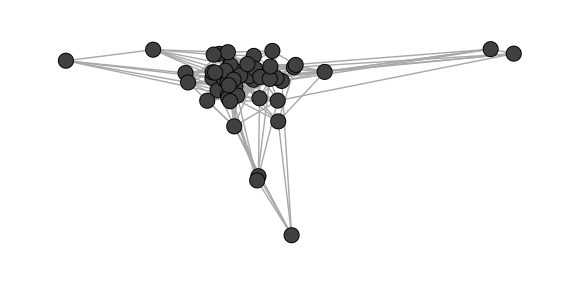# Introduction to graphlayouts

The package implements some graph layout algorithms that are not available in the igraph package.

The package implements three algorithms which serve different purposes:

• Stress majorization for general layouts (Paper)
• Quadrilateral backbone layout for (potentially) clustered networks (Paper)
• flexible radial layouts to focus on specific nodes (e.g. ego in networks) (Paper)
• spectral layouts based on eigenvectors of matrices

## Stress Majorization: Connected Network

This example is a bit of a special case since it exploits some weird issues in igraph.

library(igraph)
#>
#> Attaching package: 'igraph'
#> The following objects are masked from 'package:stats':
#>
#>     decompose, spectrum
#> The following object is masked from 'package:base':
#>
#>     union
library(ggraph)
library(graphlayouts)

set.seed(666)
pa <- sample_pa(1000,1,1,directed = F)

ggraph(pa)+
geom_node_point(col="black",size=0.3)+
theme_graph()
#> Using nicely as default layout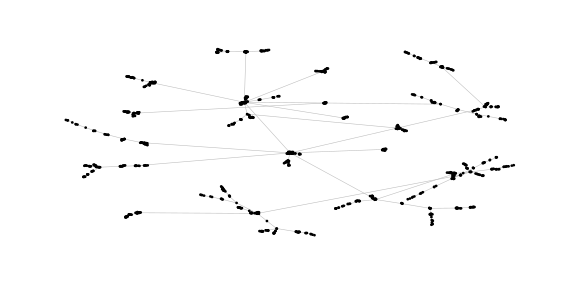ggraph(pa,layout="stress")+
geom_node_point(col="black",size=0.3)+
theme_graph()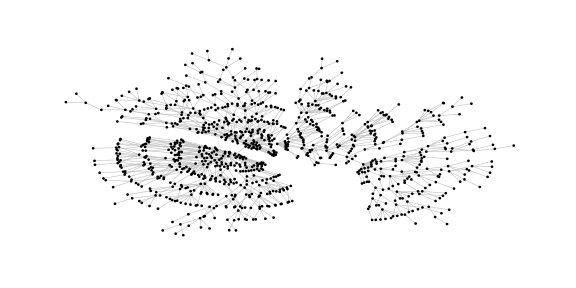## Stress Majorization: Unconnected Network

Stress majorization also works for networks with several components. It relies on a bin packing algorithm to efficiently put the components in a rectangle, rather than a circle.

set.seed(666)
g <- disjoint_union(
sample_pa(10,directed = F),
sample_pa(20,directed = F),
sample_pa(30,directed = F),
sample_pa(40,directed = F),
sample_pa(50,directed = F),
sample_pa(60,directed = F),
sample_pa(80,directed = F)
)

ggraph(g) +
geom_node_point() +
theme_graph()
#> Using nicely as default layout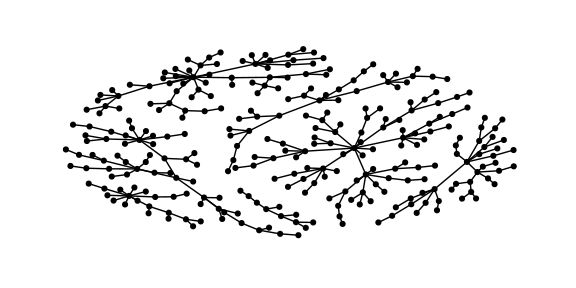ggraph(g, layout="stress") +
geom_node_point() +
theme_graph()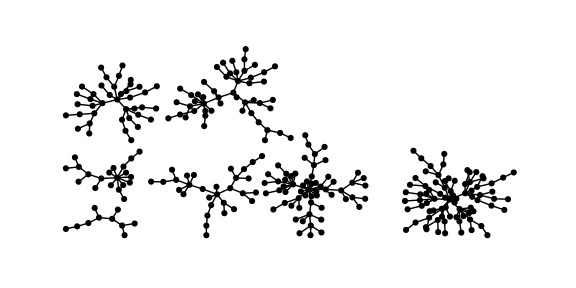## Backbone Layout

Backbone layouts are helpful for drawing so called hairballs.

set.seed(665)
#create network with a group structure
g <- sample_islands(9,40,0.4,15)
g <- simplify(g)
V(g)$grp <- as.character(rep(1:9,each=40)) ggraph(g,layout="stress")+ geom_edge_link(colour=rgb(0,0,0,0.5),width=0.1)+ geom_node_point(aes(col=grp))+ scale_color_brewer(palette = "Set1")+ theme_graph()+ theme(legend.position = "none")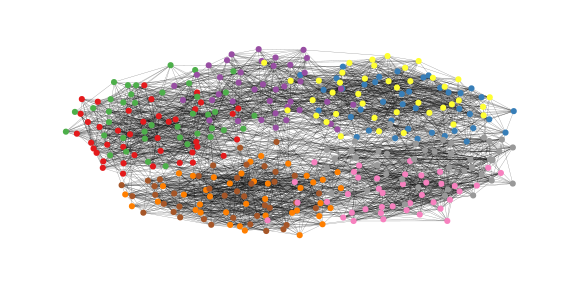The backbone layout helps to uncover potential group structures based on edge embeddedness and puts more emphasis on this structure in the layout. bb <- layout_as_backbone(g,keep=0.4) E(g)$col <- F
E(g)$col[bb$backbone] <- T

ggraph(g,layout="manual",node.positions=data.frame(x=bb$xy[,1],y=bb$xy[,2]))+
geom_node_point(aes(col=grp))+
scale_color_brewer(palette = "Set1")+
scale_edge_color_manual(values=c(rgb(0,0,0,0.3),rgb(0,0,0,1)))+
theme_graph()+
theme(legend.position = "none")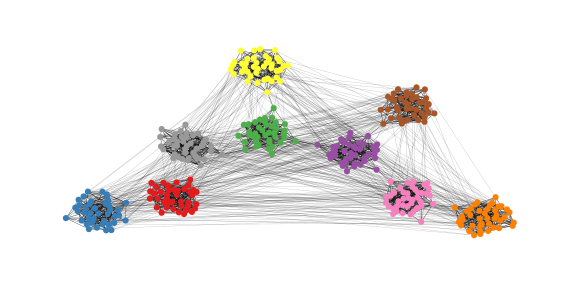## Radial Layout with Focal Node

The function layout_with_focus creates a radial layout around a focal node. All nodes with the same distance from the focal node are on the same circle.

#library(igraphdata)
#data("karate")

p1 <- ggraph(karate,layout = "focus",v = 1) +
draw_circle(use = "focus",max.circle = 3)+
geom_node_point(aes(fill=as.factor(Faction)),size=2,shape=21)+
theme_graph()+
theme(legend.position = "none")+
coord_fixed()+
labs(title= "Focus on Mr. Hi")

p2 <- ggraph(karate,layout = "focus",v = 34) +
draw_circle(use = "focus",max.circle = 4)+
geom_node_point(aes(fill=as.factor(Faction)),size=2,shape=21)+
theme_graph()+
theme(legend.position = "none")+
coord_fixed()+
labs(title= "Focus on John A.")

p1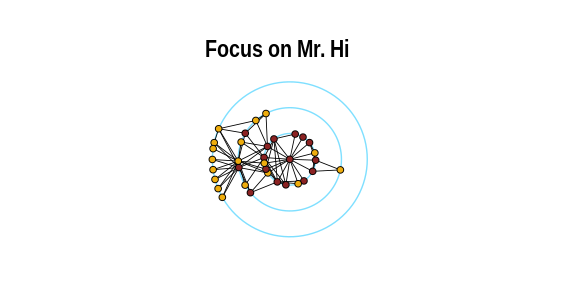p2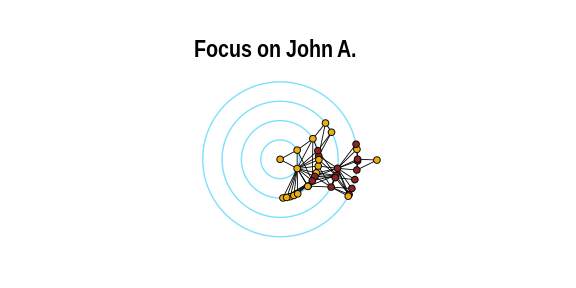The function layout_with_centrality creates a radial layout around the node with the highest centrality value. The further outside a node is, the more peripheral it is.


bc <- betweenness(karate)
p1 <- ggraph(karate,layout = "centrality", cent = bc, tseq = seq(0,1,0.15)) +
draw_circle(use = "cent") +
annotate_circle(bc,format="",pos="bottom") +
geom_node_point(aes(fill=as.factor(Faction)),size=2,shape=21)+
theme_graph()+
theme(legend.position = "none")+
coord_fixed()+
labs(title="betweenness centrality")

cc <- closeness(karate)
p2 <- ggraph(karate,layout = "centrality", cent = cc, tseq = seq(0,1,0.2)) +
draw_circle(use = "cent") +
annotate_circle(cc,format="scientific",pos="bottom") +
geom_node_point(aes(fill=as.factor(Faction)),size=2,shape=21)+
theme_graph()+
theme(legend.position = "none")+
coord_fixed()+
labs(title="closeness centrality")

p1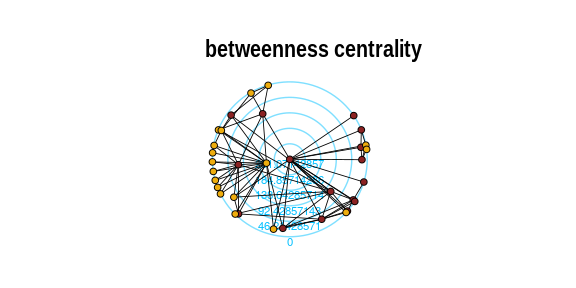p2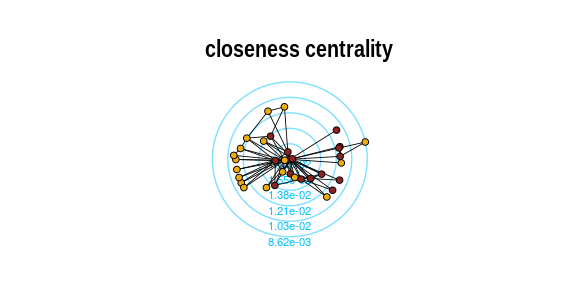## Spectral Layouts

Spectral layouts use eigenvectors of matrices associated with a graph for layouting. Possible matrices are the adjacency matrix and the laplacian matrix. Additionally, one can choose if the largest or smallest eigenvectors should be used.

g <- sample_gnp(50,0.2)

geom_node_point(shape=21,fill="grey25",size=5)+
theme_graph()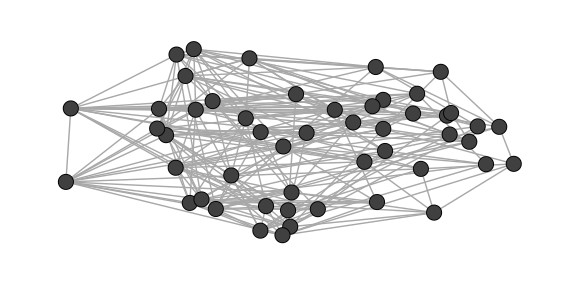geom_node_point(shape=21,fill="grey25",size=5)+
theme_graph()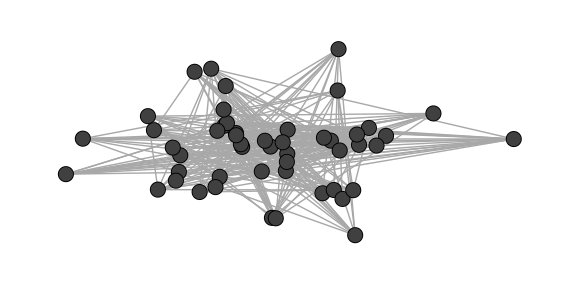ggraph(g,layout="eigen",type="laplacian",ev="largest")+
theme_graph()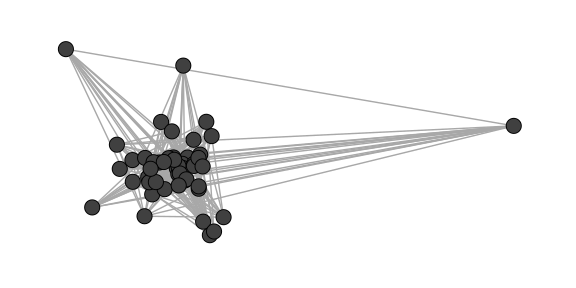theme_graph()